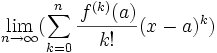## Saturday, March 11, 2006

### Taylor Series

The Taylor Series is one of the most useful results in all of mathematics. The method in all its generality was first presented by Brook Taylor. To see an application for Taylor's Series, check out Euler's Formula.

The proof for Taylor's Series depends on basic calculus.

For anyone who does not feel comfortable with the notation f(x) or the concept of a mathematical function, start here.

For anyone who does not know about derivatives or about the notation f'(x), f''(x), or fn(x), start here.

Theorem: Taylor Series
if
(a) f is a function with derivatives of all orders
(b) (lim n → inf)[f(n+1)(z)]/(n+1)!(x-a)n+1 = 0
then:
f(x) = f(a) + f'(a)(x-a) + [f''(a)/2!](x-a)2 + .... + [f(n)(a)/n!](x-a)n + ....

Proof:

(1) Let n be an arbitrary positive integer.

(2) From Taylor's Formula, we have:
f(x) = Pn(x) + Rn(x)

where:

Pn(x) = f(a) + f'(a)(x-a) + f''(a)[(x-a)2]/2! + ... + f(n)[(x-a)n]/n!

and

Rn(x) = [f(n+1)(z)]/(n+1)!(x-a)n+1

where z is between a and x.

(3) From assumption b, we see that:
lim (n → inf) Rn(x) = 0.

(4) Then it follows that:
f(x) = lim (n → inf) Pn(x) == f(a) + f'(a)(x-a) + [f''(a)/2!](x-a)2 + .... + [f(n)(a)/n!](x-a)n + ....

QED

References

## Friday, March 10, 2006

### Taylor's Formula

In today's blog, I will go over Taylor's Formula. This is a theorem that can be used to establish the Taylor Series and the MacLaurin Series which I use in my proof of Euler's Identity.

If you are not familiar with continuous functions or closed intervals, start here.

If you would like a review of derivatives, start here.

Theorem: Taylor's Formula

Let f(x) be a continuous function over the closed interval [a,b] that has an (n+1) derivative which is referenced by f(n+1)(x) where n is a positive integer.

Then:

f(b) = f(a) + f'(a)(b-a) + ... + [f(n)(a)/n!](b-a)n + [f(n+1)(z)/(n+1)!](b-a)n+1)

for some number z which lies between a and b.

Proof:

(1) Let H = f(b) - f(a) - f'(a)(b-a) - [f''(a)/2!](b-a)2 - ... - [f(n)(a)/n!](b-a)n

(2) Let K = H/(b-a)n+1

(3) Let g(x) be a function such that:
g(x) = f(b) - f(x) - f'(x)(b-x) -[f''(x)/2!](b-x)2 - ... - [f(n)(x)/n!](b-x)n - K(b-x)n+1

(4) We can see that g(x) is a continuous function since:

(a) f(x) is continuous over [a,b] by the given.

(b) f(n) is a continuous function since by the given we know that f(x) has an (n+1) derivative and since f(x) is differentiable at x, then it is continuous at x (see here)

(c) (b-x)n is continuous over [a,b] since f(x) is continuous since:

Let h(x)=b-x

h(x) is continuous since it is the addition of two continuous functions i(x)=b and j(x)=-x [See here for proof of the Addition Law]

(b-x)n is continuous by the Multiplication Law [See here for proof of the Multiplication Law]

(b-x)n = (b - x)*(b-x)*... since n is a positive integer.

(d) f(b) is continuous since it is a constant. (See here)

(e) Each f(n)(x)/n! is continuous since (-1/n!) can be thought of as a constant function h(x)=(-1/n!) and the multiplication of two continuous functions is itself continuous (see here)

(f) Finally, g(x) is continuous because the addition of a set of continuous functions is itself continuous (see here)

(5) We can see that g(a) = 0 since:

g(a) = H - H/(b-a)n+1*(b-a)n+1 = H - H = 0

(6) We can also see that g(b) = 0 since:

g(b) = f(b) - f(b) - f'(b)(b-b) - [f''(b)/2!](b-b)2 - ... - [f(n)(b)/n!](b-b)n - K(b-b)n+1

(7) By Rolle's Theorem, since g(x) is continuous on [a,b], we know that there exists a value z such that z ∈ [a,b] and g'(z) = 0. [See here for Rolle's Theorem]

(8) If we differentiate on g(x), we get:
g'(x) = -f'(x) + f'(x) -f(2)(x)(b-x) + f(2)(x)(b-x) - (1/2!)f(3)(x)(b-x)2 + (1/2!)f(3)(x)(b-x)2 - (1/3!)f(4)(x)(b-x)3 + ... + [1/(n-1)!]f(n)(x)(b-x)n-1 - (1/n!)f(n+1)(x)(b-x)n + (n+1)K(b-x)n since:

(a) g(x) = f(b) - f(x) - f'(x)(b-x) -[f''(x)/2!](b-x)2 - ... - [f(n)(x)/n!](b-x)n - K(b-x)n+1

(b) By Lemma 3 here, we can differentiate each individual product in the sum.

(c) f(b) is a constant so d/dx(f(b)) = 0 [See here for Constant Rule]

(d) d/dx(-f(x)) = -f'(x) [See here for details]

(e) d/dx[-f'(x)(b-x)] = -f''(x)(b-x) - f'(x)(-1) = f'(x) -f''(x)(b-x) [See here for the Product Rule]

(f) d/dx[(-f''(x)/2!)(b-x)2] = (-f(3)(x)/2!)(b-x)2 + [-f''(x)/2!](2)(b-x)(-1)] =
= (-f(3)/2!)(b-x)2 + f''(x)(b-x) [See here for Generalized Power Rule]

(g) d/dx([-f(n)(x)/n!](b-x)n) = (-f(n+1)(x)/n!)(b-x)n + [-f(n)(n)/n!](n)(b-x)n-1*(-1) =
= (-f(n+1)(x)/n!)(b-x)n +[ f(n)/(n-1)!](b-x)n-1

(h) Since K is a constant
d/dx(-K(b-x)n+1) = (n+1)(-K)(b-x)n(-1) = (n+1)K(b-x)n

(9) We see that most of the terms cancel out so that we get:
g'(x) = (n+1)K(b-x)n - (1/n!)f(n+1)(x)(b-x)n

(10) Applying the fact that g'(z) = 0 from step #7 gives us:
g'(z) = (n+1)K(b-z)n - (1/n!)f(n+1)(z)(b-z)n = 0

(11) We can divide both sides by (b-z)n to get:
(n+1)K - (1/n!)f(n+1)(z) = 0

(12) Now we can rearrange (#11) to get:
K = [(1/n!)f(n+1)(z)]/(n+1) = [f(n+1)(z)]/(n+1)!

(13) Now, using (#12) and (#3) with x=a, we get:
g(a) = 0 = f(b) - f(a) - f'(a)(b-a) - [f''(a)/2!](b-a)2 - ... - [f(n)(a)/n!](b-a)n - [(b-a)n+1][f(n+1)(z)/(n+1)!]

(14) Now, if we move over all the elements after f(b), we get:
f(b) = f(a) + f'(a)(b-a) + [f''(a)/2!](b-a)2 + ... + [f(n)(a)/n!](b-a)n + [(b-a)n+1][f(n+1)(z)/(n+1)!]

QED

References